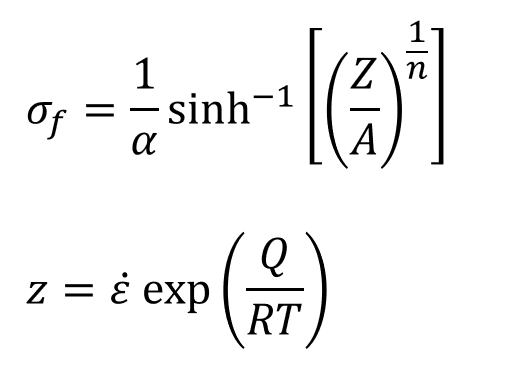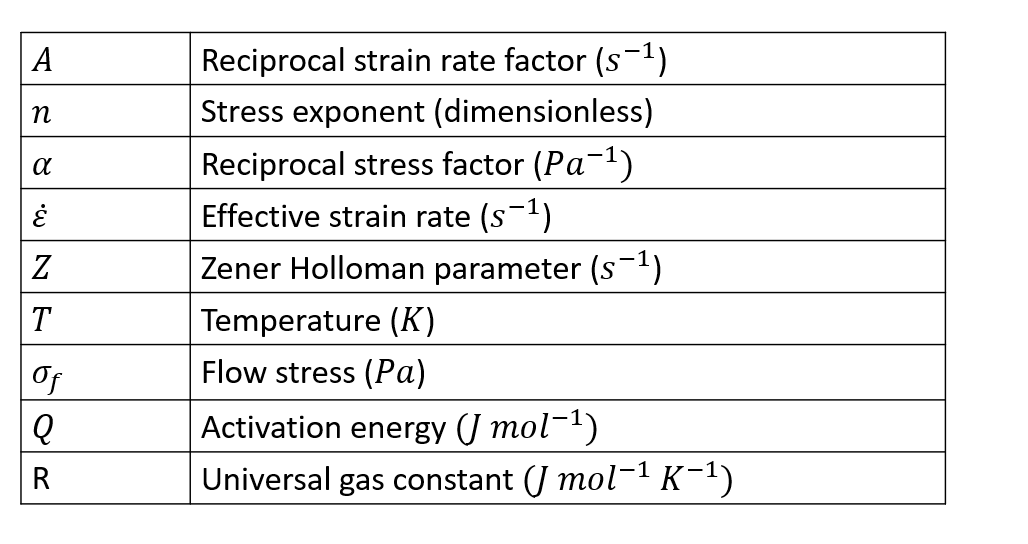# Sine Hyperbolic Inverse Model

The Sine Hyperbolic Inverse model is given by the following expression.Workpiece Aluminium { ConstitutiveModel = "SineHypINv" Density = ρ SpecificHeat = Cp (T) Conductivity = K (T) CoeffOfThermalExpansion = βT VolumericHeatSource = Qvol StressExponent = n ActivationEnergy = Q ReferenceTemperatrue T0 SolidusTemperature = Ts LiquidusTemperature = T1 ReciprocalStrainFactor = A UniversalGasConstant = R Alpha = α StrainRateOffset = ε YoungModulus = E PoissonRatio = ν}